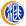## 外汇财经晚餐——你每日必备的交易攻略(9月1日)

2020-09-02 14:54 | 未知 |

9月1日，沪深两市低开高走，截至收盘，沪指涨0.44%，深证成指涨0.66%，创业板指涨0.81%。盘面上，特斯拉、无人机、军工、新能源汽车等板块涨幅居前；白酒、保险、食品饮料、疫苗等板块跌幅居前。创业板低价股再度走强，坚瑞沃能、华昌达、三丰智能、恒泰艾普、银邦股份等涨停。500)this.width=500 align=center hspace=10 vspace=10 rel=nofollow/>

500)this.width=500 align=center hspace=10 vspace=10 rel=nofollow/500)this.width=500 align=center hspace=10 vspace=10 rel=nofollow/>

500)this.width=500 align=center hspace=10 vspace=10 rel=nofollow/500)this.width=500 align=center hspace=10 vspace=10 rel=nofollow/>

500)this.width=500 align=center hspace=10 vspace=10 rel=nofollow/500)this.width=500 align=center hspace=10 vspace=10 rel=nofollow/>

500)this.width=500 align=center hspace=10 vspace=10 rel=nofollow/500)this.width=500 align=center hspace=10 vspace=10 rel=nofollow/>

500)this.width=500 align=center hspace=10 vspace=10 rel=nofollow/（责任编辑：dd）